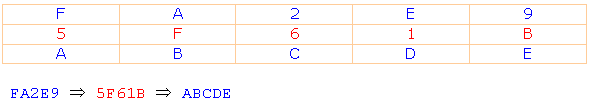Research website of Vyacheslav Gorchilin
2014-06-18
All articles
Description Hash-Mask algorithm for confidential data exchange

1. The General approach
The algorithm is based on the method of masking and demaskirovanie data (VD) for the hash mask (CMS). Khms is a hash derived from the random key (SKL), code words (CW) and number of groups (NGR).

The cryptographic strength of the Algorithm is determined by the specific its implementation depends entirely on the encryption method, hashing to create a CMS. For example, if it used MD5, then the security will get about 3.4*1038. And disadvantages of MD5, like the transmission of two messages with the same hash-sum are eliminated by the Algorithm. Even for the same input data obtained at the output of the Algorithm is the encrypted string (SSTR), each time totally different.

Method for hashing SHA-2 (SHA-256/224) the cryptographic strength of the Algorithm increases to 1077.

SSTR can be transmitted via open communication channels and requires no additional secret protocols.

2. Terms and definitions
SKL — consists of a set of hexadecimal numbers and is formed by the transferring party for each message randomly. The length of the SCR depends on the specific implementation of the Algorithm and is set only on the basis of reasonable assumptions. For example, if next will be used the MD5 hashing, then the SCR is less than 16 characters may affect the reliability of, and more than 32 characters is impractical.

COP — code word known only to the receiving and transmitting side. Its main feature is that it must be passed by independent (other) communication channels. For example, if the data transmission uses the Internet, then the COP passing through the GSM channel.

NGR is the group number. Data (VD) are divided into groups along the length of the hash mask. For example, if next will be used the MD5 hashing, the length of the group is equal to 32 bytes. Believe that VD is represented in hexadecimal form, then the total number of groups you can get VD by dividing the length by 32 and rounding the resulting number. The first group is assigned the number "0", the second "1" etc.

3. Description of the data encryption algorithm
3.1 SKL Generated a set of random hexadecimal numbers.

3.2 For the first group VD obtained hash gluing SKL, KS, and "0" for the group number. Masked data this group method . Similarly, for the second group received a hash of gluing SKL, KS, and "1", the masked data. Etc.

3.3 Bonding of the SCR and masked data of all groups in a string (SSTR), which can transmit on open channels of communication.

4. Description of the algorithm decrypt data
4.1 obtained From the SSTR detachable SKL whose length is known. Divide the remaining data into groups.

4.2 For the first group of the data received hash gluing SKL, KS, and "0" for the group number. Demirkiran data of this group method . Similarly, for the second group received a hash of gluing SKL, KS, and "1", demirkiran data. Etc.

4.3 Bonding unmasked data of all the groups and obtain an initial VD of claim 3.

The implementation of the algorithm | is Another implementation of the algorithm

 We will use a fairly simple and quick masking algorithm. It is based on the addition of two hexadecimal numbers after which it remains one the youngest category, for example: A+5=F C+6=2. As a result of transformation get the String FA2E9, the length of which is equal to the length of VD. An example of algebraic transformation shown in Fig.1, where VD is equal to ABCDE and equal khms 5F61B.Fig.1

 All operations reverse the operations in the example from . Need of value data element, subtract the value of the item khms method Supplement. Ie if first number is less than another, then the obtained difference is added the number corresponding to the numeral system (for example the hexadecimal number system is 16).Fig.2